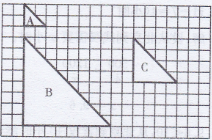# 人教版六年级下册数学书练习十一答案

D

（比例不唯一）(1)三兔形B和三角形C是三角形A放大后得到的图形。

(2)三角形A和三角形C是三角形B缩小后得到的图形。

(3)分析：三角形A的面积是2×2×1/2=2，三角形B的面积是8×8×1/2=32，

2.4/1.5=4/x x=2.5

1.6/6=x/14 x=371/15

8x=6×12 x=9

11-7=4(小时)

500/4=1200/x

x=9.6

9.6<10，从北京到武汉10小时能到。